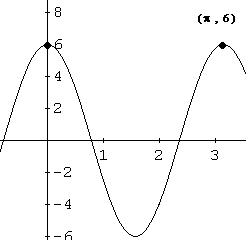# Tutorial on Sine Functions (1)- Problems

This is a tutorial on sine function problems. Examples with detailed solutions and explanations are included.

## Example - Problem 1

Find a, b and c included in the definition the sine function f given by

f(x) = a*sin(bx + c)

such that the maximum value of f(x) is 6, f(0) = 6 and the period of the graph of function f is equal to pi. a, b and c are positive and c is less than 2pi.

Solution to Problem 1:

1. The maximum value 6 gives the amplitude
| a | = 6

2. Solve the above equation for a and select the positive value.
a = 6

3. The period may be used to find b using
period = 2*pi / | b | = pi

4. Solve for | b |
| b | = 2

5. Solve for b and select the positive value
b = 2

6. Substitute 6 for a in the formula of the function and use f(0) = 6 to write an equation in c.
6 = 6*sin(b*0 + c)

7. Which gives sin(c) = 1

8. Solve for c
c = pi/2 + k(2*pi) , where k is an integer.
There is an infinite number of solutions for c.

9. Select k = 0 since it is the only value that gives c > 0 and less that 2*pi, which gives
c = pi / 2

10. Function f is given by f(x) = 6*sin(2x + pi/2)

11. For checking, part of the graph of f is shown below. Check the period and f(0) = 6 and that the maximum value of f(x) is 6.Matched Problem 1: Find a, b and c in the sine function f given by

f(x) = a*sin(bx + c)

such that the minimum value of f is -3, f(0) = -3 and the period of the graph of the function is equal to 4*pi. a, b and c are positive and c is less than 2 pi.